• C程序设计谭浩强第五版课后答案 第四章习题答案 scanf出问题请看：超简单的scanf错误修改 4. 有3个整数a, b, c，由键盘输入，输出其中最大的数。 #define _CRT_SECURE_NO_WARNINGS #include <stdio.h> int ...
C程序设计谭浩强第五版课后答案 第四章习题答案
scanf出问题请看：超简单的scanf错误修改
4. 有3个整数a, b, c，由键盘输入，输出其中最大的数。
#define _CRT_SECURE_NO_WARNINGS
#include <stdio.h>
int main()
{
int a, b, c;
scanf("%d %d %d", &a, &b, &c);
if (a == b && a == c) {
printf("Three numbers are equal\n");
}else if (a == b && a > c) {
printf("a and b are the largest number\n", a);
}else if (a == c && a > b) {
printf("a and c are the largest number\n", a);
}else if (b == c && b > a) {
printf("c and b are the largest number\n", a);
}else if (a > b && a > c) {
printf("a=%d is the largest number\n", a);
}else if (b > a && b > c) {
printf("b=%d is the largest number\n", b);
}else {
printf("c=%d is the largest number\n", c);
}
return 0;
}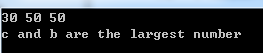5.从键盘输入一个小于1000的正数,要求输出它的平方根(如平方根不是整数，则输出其整数部分)。要求在输入数据后先对其进行检查是否为小于1000 的正数。若不是,则要求重新输入。
#include <stdio.h>
#include <math.h>
int main()
{
float a, b;
scanf_s("%f", &a);
if (a >= 1000 || a < 0) {
printf("请输入小于1000的正数\n");
scanf_s("%f", &a);
b = sqrt(a);
}
else {
b = sqrt(a);
}
printf("a=%4.0f, b=%4.0f\n", a, b);
system("pause");//这一句是为了让控制台不退出
return 0;
}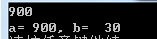6. 有一个函数，编写程序，输入x的值，输出y相应的值。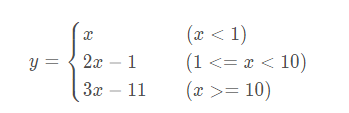#define _CRT_SECURE_NO_WARNINGS
#include <stdio.h>
int main()
{
int x, y;
scanf("%d", &x);
if (x < 1)
{
y = x;
}
else if (x >= 1 && x < 10)
{
y = 2 * x - 1;
}
else
{
y = 3 * x - 11;
}
printf("y = %d\n", y);
//system("pause");//控制台没设置好的，加上这个或者设置控制台
return 0;
}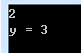7. 有一个函数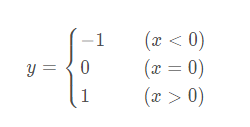有人编写了以下两个程序,请分析它们是否能实现题目要求。不要急于上机运行程序,先分析两个程序的逻辑,画出它们的流程图，分析它们的运行情况。然后，上机运行程序,观察和分析结果。
#include <stdio.h>

int main()
{
int x, y;
printf("enter x:");
scanf("%d", &x);
y=0;
if (x >= 0)
if (x > 0) y=1;
else y=-1;
printf("x=%d,y=%d\n", x, y);
return 0;
}


8. 给出一百分制成绩,要求输出成绩等级’A’、‘B’、‘C’、‘D’、‘E’。 90分以上为’A’,8089分为’B’,7079分为’C’ ,60~69分为’D’ ,60分以下为’E’。
#include <stdio.h>
int main()
{
int score;
printf("enter score:");
scanf_s("%d", &score);
if (score >= 90) {
printf("A\n");
}else if (score >= 80 && score < 90) {
printf("B\n");
}else if (score >= 70 && score < 80) {
printf("C\n");
}else if (score >= 60 && score < 70) {
printf("D\n");
}else {
printf("E\n");
}
system("pause");
return 0;
}


9. 给一个不多于5位的正整数,要求:①求出它是几位数;②分别输出每一位数字;③按逆序输出各位数字，例如原数为321,应输出123。
解（1）
#include <stdio.h>
int main()
{
int num;
printf("enter num:");
scanf_s("%d", &num);
if (num > 99999 || num < 0) {
printf("请输入0~99999之间的正数\n");
return -1;
}
if (num >= 10000) {
printf("5\n");
}else if (num >= 1000) {
printf("4\n");
}else if (num >= 100) {
printf("3\n");
}else if (num >= 10) {
printf("2\n");
}else {
printf("1\n");
}
system("pause");
return 0;
}


解（2）
#include <stdio.h>
int main()
{
int num;
printf("enter num:");
scanf_s("%d", &num);
if (num > 99999 || num < 0) {
printf("请输入0~99999之间的数字\n");
return -1;
}
if (num / 10000 > 0) {//取出万位数字
printf("%d ", num / 10000);
}
if (num%10000 >= 1000) {//取余10000则可以取出低四位的数据，除以1000则得到千位的数字
printf("%d ", (num % 10000) / 1000);
}
if (num%1000 >= 100) {//取余1000则可以取出低三位的数据，除以100则得到百位的数字
printf("%d ", (num % 1000) / 100);
}
if (num%100 >= 10) {//取余100则可以取出低两位的数据，除以10则得到十位的数字
printf("%d ", (num % 100) / 10);
}
if (num%10 >= 0) {//取余10则取出个位数字
printf("%d ", num % 10);
}
printf("\n");
system("pause");
return 0;
}


解（3）
#include <stdio.h>
int main()
{
int num;
printf("enter num:");
scanf_s("%d", &num);
if (num > 99999 || num < 0) {
printf("请输入0~99999之间的数字\n");
return -1;
}
if (num % 10 >= 0) {
printf("%d ", num % 10);
}
if (num % 100 >= 10) {
printf("%d ", (num % 100) / 10);
}
if (num % 1000 >= 100) {
printf("%d ", (num % 1000) / 100);
}
if (num % 10000 >= 1000) {
printf("%d ", (num % 10000) / 1000);
}
if (num / 10000 > 0) {
printf("%d ", num / 10000);
}
printf("\n");
system("pause");
return 0;
}


10.企业发放的奖金根据利润提成。利润I低于或等于100000元的,奖金可提成10%；利润高于100000元，低于200000元(100000<I≤200000)时,低于100000元的部分按10%提成,高于100000元的部分,可提成7. 5%；200000<I≤400000时，低于200000元的部分仍按上述办法提成(下同)。高于200000元的部分按5%提成；400000<<I≤600000元时，高于400000元的部分按3%提成；600000<1≤1000000时,高于600000元的部分按1.5%提成；I>1000000时,超过1000000元的部分按1%提成。从键盘输入当月利润I,求应发奖金总数。要求：(1) 使用if语句编写程序。(2) 使用switch语句编写程序。
解（1）
#include <stdio.h>
int main()
{
double I, salary = 0;
printf("enter performance:");
scanf_s("%lf", &I);
if (I < 0) {
printf("请输入一个正数\n");
system("pause");
return -1;
}
double salary1 = 100000 * 0.1;//10万的奖金
double salary2 = (200000 - 100000) * 0.075 + salary1;//20万的奖金
double salary3 = (400000 - 200000) * 0.05 + salary2;//40万的奖金
double salary4 = (600000 - 400000) * 0.03 + salary3;//60万的奖金
double salary5 = (1000000 - 600000) * 0.015 + salary4;//100万的奖金
if (I <= 100000) {
salary = I * 0.1;//小于100000按10%提成
}else if (I > 100000 && I <= 200000) {
salary = salary1 + (I - 100000) * 0.075;//多出10万的按比例计算，加上10w的奖金
}else if (I > 200000 && I <= 400000) {
salary = salary2 + (I - 200000) * 0.05;//多出20万的按比例计算，加上20w的奖金
}else if (I > 400000 && I <= 600000) {
salary = salary3 + (I - 400000) * 0.03;//多出40万的按比例计算，加上40w的奖金
}else if (I > 600000 && I <= 1000000) {
salary = salary4 + (I - 600000) * 0.015;//多出60万的按比例计算，加上60w的奖金
}else if (I > 1000000){
salary = salary5 + (I - 1000000) * 0.01;//多出100万的按比例计算，加上100w的奖金
}
printf("salary:%f\n", salary);
system("pause");
return 0;
}


解（2）
#include <stdio.h>
int main()
{
double I, salary = 0;
printf("enter performance:");
scanf_s("%lf", &I);
if (I < 0) {
printf("请输入一个正数\n");
system("pause");
return -1;
}
double salary1 = 100000 * 0.1;//大于100000时0~100000的奖金
double salary2 = (200000 - 100000) * 0.075 + salary1;//大于200000时0~20万的奖金
double salary3 = (400000 - 200000) * 0.05 + salary2;//大于400000时0~40万的奖金
double salary4 = (600000 - 400000) * 0.03 + salary3;//大于600000时0~60万的奖金
double salary5 = (1000000 - 600000) * 0.015 + salary4;//大于1000000时0~100万的奖金
int grade = I / 100000;
switch(grade) {
case 0:
salary = I * 0.1; break;
case 1:
salary = salary1 + (I - 100000) * 0.075; break;
case 2://会顺序执行到下一个break处
case 3:
salary = salary2 + (I - 200000) * 0.05; break;
case 4:
case 5:
salary = salary3 + (I - 400000) * 0.03; break;
case 6:
case 7:
case 8:
case 9:
salary = salary4 + (I - 600000) * 0.015; break;
default:
salary = salary5 + (I - 1000000) * 0.01; break;
}
printf("salary:%f\n", salary);
system("pause");
return 0;
}


11. 输入4个整数，要求按由小到大的顺序输出。
#include <stdio.h>
int main()
{
int a, b, c, d;
int max_num;
scanf_s("%d %d %d %d", &a, &b, &c, &d);
int tmp;
//找到最小的数
if (a > b) {
tmp = a; a = b; b = tmp; // a>b两个数据交换,则给a存储小的b
}
if (a > c) {
tmp = a; a = c; c = tmp;
}
if (a > d) {
tmp = a; a = d; d = tmp;
}
//找到第二小的数，不需要和最小的数比较
if (b > c) {
tmp = b; b = c; c = tmp;
}
if (b > d) {
tmp = b; b = d; d = tmp;
}
//找到第三小的数据，不需要和第一和第二小比较
if (c > d) {
tmp = c; c = d; d = tmp;
}
printf("%d %d %d %d\n", a, b, c, d);
system("pause");
return 0;
}


12. 有4个圆塔,圆心分别为(2,2)、(-2,2)、(-2,-2)、(2,-2),圆半径为1。这4个塔的高度为10m,塔以外无建筑物。今输入任一点的坐标，求该点的建筑高度(塔外的高度为零)。
#include <stdio.h>
#include <math.h>
void main()
{
int h;
double x, y, m, n, r;
printf("Please input a coordinate (x,y):");
scanf_s("%lf,%lf", &x, &y);
if (fabs(x) > 3 || fabs(y) > 3) {
h = 0;
printf("The height of the coordinate(%f,%f):h=%d\n", x, y, h);
return 0;
}
m = fabs(x) - 2; n = fabs(y) - 2;
r = sqrt(m * m + n * n);
if (r > 1)
h = 0;
else
h = 10;
printf("The height of the coordinate(%f,%f):h=%d\n", x, y, h);
system("pause");
return 0;
}




展开全文程序设计 c语言
• 算术运算即“则运算”，是加法、减法、乘法和除法种运算的统称。它通常是对实数或复数进行的 关系运算就是“比较运算”，将两个数值进行比较，判断其比较的结果是否符合给定的条件。 逻辑运算是将多个变量或者...
1.什么是算术运算？什么是关系运算？什么是逻辑运算？
算术运算即“四则运算”，是加法、减法、乘法和除法四种运算的统称。它通常是对实数或复数进行的
关系运算就是“比较运算”，将两个数值进行比较，判断其比较的结果是否符合给定的条件。
逻辑运算是将多个变量或者表达式链接起来的逻辑关系
2.C语言中如何表示“真”和“假”？系统如何判断一个量的“真”和“假”？
C语言中将数值为1作为真，为0作为假，系统将值为非0的变量或表达式作为真，否则假。
3.写出下面各逻辑表达式的值。设a=3,b=4,c=5.
（1）a+b>c&&b==c
（2）a||b+c&&b-c   //短路现象，针对逻辑或
（3）!(a>b)&&!c||1
（4）!(x=a)&&(y=b)&&0    //短路
（5）!(a+b)+c-1&&b+c/2
即：（1）0 因为b!=c;
（2）1 因为a,b+c,b-c均非0；
（3）1 因为||右侧为1 逻辑运算符||其中一个表示为1值则可以判定；
（4）0 很明显第一个和第三个式子为假 &&其中一个为假 则判定；
（5）1 &&前可以表示为0+5-1，后表示4+5/2均非0 。
4.有3个整数，由键盘输入，输出其中最大的数。
  #include<stdio.h>
int main()
{
int a,b,c,z;
printf("请输入三个数\n");
scanf("%d %d %d",&a,&b,&c);
if(a>b){
z=a;
}else{
z=b;
}if(c<b||(c>b&&c<a)){
z=a;
}else{
z=c;
}
printf("max=%d",z);
return 0;
}

5.从键盘输入一个小于1000的正整数，要求输出它的平方根（如平方根不是整数，则输出其整数部分）。要求在输入数据后先对其检查是否为小于1000的正数。若不是，则要求从新输入。
#include <stdio.h>
#include <math.h>
int main()
{
int i,k;
int m=1000;
printf("请输入一个小于%d的整数i:",m);
scanf("%d",&i);
if (i>m) {
printf("输入的数不符合要求，请重新输入一个小于1000的整数i:\n");
scanf("%d",&i);
}
k=sqrt(i);
printf("%d的平方根的整数部分是：%d\n",i,k);
return 0;
}

6.有一个函数：
{x (x<1)
y={2x-1 (1<=x<10)
{3x-11 (x>=10)
写程序，输入x的值，输出y相应的值。
#include<stdio.h>
int main(){
int x,y;
printf("请输入x的值：");
scanf("%d",&x);
if(x<1){
y=x;
}else if(x>1&&x<10){
y=2*x-1;
}else{
y=3*x-11;
}
printf("x=%d,y=%d",x,y);
return 0;
}

7.参照6.
8.给出一个百分制成绩，要求输出成绩等级A,B,C,D,E。90分以上为A,8089分为B,7079分为C,60~69分为D,60分以下为E。
#include<stdio.h>
int main()
{
int score;
printf("输入学生的成绩:\n");
scanf("%d",&score);
if (score<0 || score>100){
printf("输入的数据非法!\n");
}else{
if(score>90){
printf("该同学的等级为:A");
}
if(score>=80 && score<=90){
printf("该同学的等级为:B");
}
if(score>=70 && score<=79){
printf("该同学的等级为:C");
}
if(score>=60 && score<=69){
printf("该同学的等级为:D");
}
if(score>=0&&score<=59){
printf("该同学的等级为:E");
}
}
return 0;
}

9.给出一个不多于5位数的正整数；
（1）求出它是几位数；
（2）分别输出每一位数字；
（3）按逆顺序输出各位数，例如原有数为123，应输出321
#include<stdio.h>
main()
{
long num;
printf("输入一个不多于5位的整数:\n");
scanf("%ld",&num);
int place;
if(num>9999) place=5;
else if(num>999) place=4;
else if(num>99) place=3;
else if(num>9) place=2;
else place=1;
printf("\n%d是%d位数\n",num,place);

int wan,qian,bai,shi,ge;
ge=num%10;
shi=num/10%10;
bai=num/100%10;
qian=num/1000%10;
wan=num/10000;
printf("\n分别打印出每一位数字和逆序输出:\n");
switch(place)
{
case 5:printf("%d\t%d%d%d%d%d\n",num,ge,shi,bai,qian,wan);break;
case 4:printf("%d\t%d%d%d%d\n",num,ge,shi,bai,qian);break;
case 3:printf("%d\t%d%d%d\n",num,ge,shi,bai);break;
case 2:printf("%d\t%d%d\n",num,ge,shi);break;
case 1:printf("%d\t%d\n",num,ge);break;
}
}


#include<stdio.h>
main()
{
long i;
float bonus,bon1,bon2,bon4,bon6,bon10;
int c;
bon1=10000*0.1;
bon2=bon1+100000*0.075;
bon4=bon2+200000*0.05;
bon6=bon4+200000*0.03;
bon10=bon6+400000*0.015;
printf("请输入利润i:");
scanf("%ld",&i);
c=i/100000;
if(c>10) c=10;
switch(c)
{
case 0:bonus=1*0.1;break;
case 1:bonus=bon1+(i-100000)*0.075;break;
case 2:
case 3:bonus=bon2+(i-200000)*0.05;break;
case 4:
case 5:bonus=bon4+(i-400000)*0.03;break;
case 6:
case 7:
case 8:
case 9:bonus=bon6+(i-600000)*0.015;break;
case 10:bonus=bon10+(i-1000000)*0.01;break;
}
printf("奖金是%10.2f",bonus);
}

11.输入4个整数，要求按由小到大的顺序输出。
  方法一：
#include<stdio.h>
int main()
{
int a,b,c,d,t;
printf("请输入四个整数：\n");
scanf("%d%d%d%d",&a,&b,&c,&d);
if(a>b)
{
t=a;a=b;b=t;
}
if(a>c)
{
t=a;a=c;c=t;
}
if(a>d)
{
t=a;a=d;d=t;
}
if(b>c)
{
t=b;b=c;c=t;
}
if(b>d)
{
t=b;b=d;d=t;
}
if(c>d)
{
t=c;c=d;d=t;
}
printf("%d%d%d%d",a,b,c,d);
}

方法二数组
   #include <stdio.h>

int main()
{
int a ;
int i,j;
int temp;
printf("请输入4个数\n： ");
for( i = 0; i < 4; i++ )
{
scanf("%d",&a[i]);
}
for( i = 0; i < 4; i++ )
{
for( j = 0; j < (4-i); j++ )
if( a[j] > a[j+1] )
{
temp = a[j];
a[j] = a[j+1];
a[j+1] = temp;
}
}
printf("这4个数由小到大的排列顺序如下：");
for( i = 0; i < 4; i++ )
{
printf("%d ",a[i]);
}
printf("\n");
return 0;
}

12.有4个圆塔，圆心分别为（2,2）、（-2,2）、（-2，-2）、（2，-2），圆半径为1。这4个塔的高度为10m，塔以外无建筑物。今输入任一点的坐标，求该点的建筑高度（塔外的高度为0）
#include <stdio.h>
#include<math.h>
main()
{ int h=10;
float x1=2,y1=2,x2=-2,y2=2,x3=-2,y3=-2,x4=-2,y4=-2,x,y,d1,d2,d3,d4;
printf("请输入一个点(x,y)：");
scanf("%f,%f",&x,&y);
d1=sqrt((x-x1)*(x-x1)+(y-y1)*(y-y1)); /*求该点到各中心点的距离*/
d2=sqrt((x-x2)*(x-x2)+(y+y2)*(y+y2));
d3=sqrt((x+x3)*(x+x3)+(y-y3)*(y-y3));
d4=sqrt((x+x4)*(x-x4)*(y+y4)*(y+y4));
if(d1>1&&d2>1&&d3>1&&d4>1) h=0; /*判断该点是否在塔外*/
printf("该点高度为%d\n",h);
}



展开全文• 想看更多算法题，可以扫描上方二维码关注我微信公众号“数据结构和算法”，截止到目前我已经在公众号中更新了500多道算法题，其中部分已经整理成了pdf文档，截止到目前总共有800多页（并且还会不断的增加），可以在...想看更多算法题，可以扫描上方二维码关注我微信公众号“数据结构和算法”，截止到目前我已经在公众号中更新了500多道算法题，其中部分已经整理成了pdf文档，截止到目前总共有800多页（并且还会不断的增加），可以在公众号中回复关键字“pdf”即可下载。展开全文• c++面向对象程序设计 谭浩强 答案第一章 ...c++面向对象程序设计 谭浩强 答案第四章 c++面向对象程序设计 谭浩强 答案第五章 第1章C++ 的初步知识 1．请根据你的了解，叙述C++ 的特点。C++ 对C有哪些发展？...
c++面向对象程序设计 谭浩强 答案 第一章
目录：
c++面向对象程序设计 谭浩强 答案 第一章
c++面向对象程序设计 谭浩强 答案 第二章
c++面向对象程序设计 谭浩强 答案 第三章
c++面向对象程序设计 谭浩强 答案 第四章
c++面向对象程序设计 谭浩强 答案 第五章

第1章  C++ 的初步知识
1．请根据你的了解，叙述C++ 的特点。C++ 对C有哪些发展？
【解】 略。
2．一个C++的程序是由哪几部分构成的？其中的每一部分起什么作用？
【解】 略。
3．从拿到一个任务到得到最终结果，一般要经过几个步骤？
【解】 略。
4．请说明编辑、编译、连接的作用。在编译后得到的目标文件为什么不能直接运行？
【解】 编译是以源程序文件为单位进行的，而一个完整的程序可能包含若干个程序文件，在分别对它们编译之后，得到若干个目标文件（后缀一般为.obj），然后要将它们连接为一个整体。此外，还需要与编译系统提供的标准库相连接，才能生成一个可执行文件（后缀为.exe）。不能直接运行后缀为.obj的目标文件，只能运行后缀为.exe的可执行文件。
谭浩强 c++面向对象程序设计 课后答案
5．分析下面程序运行的结果。

1 #include <iostream>
2 using namespace std;
3 int main()
4 {
5     cout << "This" << "is";
6     cout << "a" << "C++";
7     cout << "program." << endl;
8     return 0;
9 }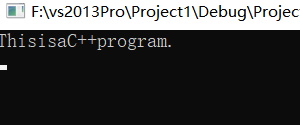【解】 输出的结果为

ThisisaC++program.

谭浩强
6．分析下面程序运行的结果。

1 #include <iostream>
2 using namespace std;
3 int main()
4 {
5     int a, b, c;
6     a = 10;
7     b = 23;
8     c = a + b;
9     cout << "a+b=";
10     cout << c;
11     cout << endl;
12     return 0;
13 }

【解】 前两个cout语句在输出数据后不换行，第3个cout语句输出一个换行，因此输出的结果为

a+b=33

7．分析下面程序运行的结果。请先阅读程序写出程序运行时应输出的结果，然后上机运行程序，验证自己分析的结果是否正确。以下各题同。

#include <iostream>

using namespace std;

int main( )

{

int a,b,c;

int f(int x,int y,int z);

cin>>a>>b>>c;

c=f(a,b,c);

cout<<c<<endl;

return 0;

}

int f(int x,int y,int z)

{

int m;

if (x<y) m=x;

else m=y;

if (z<m) m=z;

return(m);

}

c++面向对象程序设计
【解】 程序的作用是：输入3个整数，然后输出其中值最小的数。在主函数中输入3个整数，然后调用f函数，在f函数中实现找最小的整数，用if语句比较两个数，将小者存放在变量m中，经过两个if语句的比较，m中存放的是3个整数中最小的数。运行情况如下：

1 5 3↙                        (输入3个整数)
1                             (输出其中最小的数)

8．在你所用的C++系统上，输入以下程序，进行编译，观察编译情况，如果有错误，请修改程序，再进行编译，直到没有错误，然后进行连接和运行，分析运行结果。

int main( );
{
int a,b;
c=a+b;
cout >>" a+b=" >> a+b;
}

【解】 上机编译出错，编译出错信息告知在第2行出错，经检查，发现第1行的末尾多了一个分号，编译系统无法理解第2行的花括号，导致报告第2行出错。将第1行的末尾的分号去掉，重新编译，编译出错信息告知在第5行和第6行出错。第5行出错原因是cout未经声明，因为cout不是C++语言提供的系统的关键字，而是输出流的对象，必须使用头文件iostream。第6行出错原因是main是int型函数，应返回一个整型值。将程序改为

#include <iostream>
int main( )
{
int a,b;
c=a+b;
cout >>" a+b=" >> a+b;
return 0;
}

重新编译。编译出错信息告知在第5行和第6行出错。第5行出错原因是变量c未定义，第6行出错原因是cout未经声明，说明#include <iostream>命令行未能起作用，原因是未指明命名空间。将程序改为

#include <iostream>
using namespace std;
int main( )
{
int a,b,c;
c=a+b;
cout>>" a+b=" >>a+b;
return 0;
}
重新编译。编译出错信息告知在第7行出错，经检查，是“>>”用得不当，“>>”是提取运算符，应与cin联合使用，用来从输入流中提取数据，输出时应该用插入运算符“<<”。把两处“>>”都改为“<<”，再重新编译，发现没有error错误，但有两个警告（warning），原因是定义了a和b，但未对它们赋值。应增加赋值语句或输入语句，使a和b获得值，将程序改为

#include <iostream>
using namespace std;
int main( )
{
int a,b,c;
cin>>a>>b;
c=a+b;
cout>>" a+b=" >>a+b;
return 0;
}

重新编译，没有编译错误，能通过编译和连接，可以正常运行，在Visual C++ 6.0环境下运行时屏幕显示如下：

5 9↙
a+b=14Press any key to continue

显然这样的输出不理想，“Press any key to continue”是Visual C++系统在输出了运行结果后自动显示的一个信息，告诉用户“如果想继续工作，请按任何一个键”。当用户按任何一个键后，显示运行结果的窗口消失，屏幕显示回到Visual C++的主窗口，显示出源程序和编译信息。
为了解决以上输出不理想的情况，可以在最后一个输出语句中增加输出一个换行符。最后的程序如下：

#include <iostream>
using namespace std;
int main( )
{
int a,b,c;
cin>>a>>b;
c=a+b;
cout<<"a+b="<<a+b<<endl;
return 0;
}

运行时屏幕显示如下：

5 9↙
a+b=14
Press any key to continue

这就完成了程序的调试。
这里对本题的调试过程作了比较详细的分析，以便使读者对如何调试程序有比较具体而清晰的了解。需要说明：
（1）编译系统给出的编译出错信息，只是提示性的，引导用户去检查错误，用户必须根据程序的上下文和编译出错信息，全面考虑，找出真正出错之处。例如编译出错信息通知第2行出错，其实可能是第1行出错。
（2）有时，有的错误开始时未被检查出来并告知用户（例如未定义变量c），由于其他错误未解决，掩盖了这个错误。当解决了其他错误后，这个错误会被检查出来。有时在调试过程中会不断检查出新的错误，这是不奇怪的。一一处理，问题会迎刃而解。
（3）为了说明调试过程，这里全部依靠计算机系统来检查错误，其实有些明显的错误，完全可以由人工查出，这样可以提高调试效率。由人工在纸面或屏幕上检查错误，称为静态查错，用计算机编译系统检查错误，称为动态查错。建议尽量先用静态查错的方法排除错误，只有人工检查不出来的错误才让计算机检查。
9．输入以下程序，进行编译，观察编译情况，如果有错误，请修改程序，再进行编译，直到没有错误，然后进行连接和运行，分析运行结果。

#include <iostream>
using namespace std;
int main( )
{
int a,b;
c=add(a,b)
cout<<"a+b="<<c<<endl;
return 0;
}
int add(int x,int y);
{
z=x+y;
retrun(z);
}

【解】 发现7个错误：
（1）对add函数未声明就调用，应在main函数中对add函数进行声明。
（2）定义add函数时，函数首行末尾不应有分号。
（3）变量c未经定义。
（4）add函数中的变量z未经定义。
（5）第6行末尾少了一个分号。
（6）add函数中的retrun拼写错误，应为return。编译系统把retrun作为未声明的标识符而报错，因为retrun（z）会被认为是函数调用的形式。
（7）变量a和b未被赋值。
改正后的程序如下：

#include <iostream>
using namespace std;
int main( )
{int add(int x,int y);
int a,b,c;
cin >> a >> b;
c=add(a,b);
cout <<" a+b=" << c <<endl;
return 0;
}

int add(int x,int y)
{int z;
z=x+y;
return(z);
}

运行情况如下：

5 8↙
13

10．输入以下程序，编译并运行，分析运行结果。

#include <iostream>
using namespace std;
int main( )
{ void sort(int x,int y,int z);
int x,y,z;
cin >> x >> y >> z;
sort(x,y,z);
return 0;
}
void sort(int x, int y, int z)
{
int temp;
if (x>y) {temp=x;x=y;y=temp;}      //{ }内3个语句的作用是将x和y的值互换
if (z<x)  cout << z << ',' << x << ',' << y << endl;
else if (z<y) cout << x <<',' << z << ',' << y << endl;
else cout << x << ',' << y << ',' << z << endl;
}

请分析此程序的作用。sort函数中的if语句是一个嵌套的if语句。
运行时先后输入以下几组数据，观察并分析运行结果。

① 3  6  10↙
② 6  3  10↙
③ 10  6  3↙
④ 10，6，3↙

【解】 程序的作用是对输入的3个整数按由小到大的顺序进行排序。sort函数中的第1个if语句的作用是先将x和y排序，使x小于或等于y。第2个if语句是一个嵌套的if语句，先比较z和x，如果z<x，显然由小到大的顺序应当是z,x,y，按此顺序输出；如果z不小于x，而小于y，显然由小到大的顺序应当是x,z,y，按此顺序输出；如果z既不小于x，又不小于y，显然由小到大的顺序应当是x,y,z，按此顺序输出。
按题目要求分别输入以下几组数据，运行结果如下：

① 3  6  10↙
3，6，10
② 6  3  10↙
3，6，10
③ 10  6  3↙
3，6，10
④ 10，6，3↙
-858993460，-858993460，10

以上是在Visual C++ 6.0环境下运行的情况，前3次运行正常，表明当输入不同的数据时，程序能实现由小到大的排序功能。第4次运行的结果显然不正常，这是由于输入数据时出了问题，本来要求在输入数据时，数据之间以空格或换行相隔，而现在却以逗号相隔，只有第一个整数能正常赋给变量x，第二和第三个数据均无法正常赋给变量y和z，y和z的值来自输入流中相应字节的内容。
11．求2个或3个正整数中的最大数，用带有默认参数的函数实现。
【解】 可以编写出以下程序：

#include <iostream>
using namespace std;
int main( )
{int max(int a,int b,int c=0);
int a,b,c;
cin >> a >> b >> c;
cout << " max(a,b,c)= " << max(a,b,c) << endl;
cout << " max(a,b)= " <<max(a,b) << endl;
return 0;
}

int max(int a,int b,int c)
{if(b>a) a=b;
if(c>a) a=c;
return a;
}

运行情况如下：

13  5  76↙
max(a，b，c)=76                  （从3个数中找最大者）
max(a，b)=13                     （从前2个数中找最大者）

如果想从3个数中找大者，可以在调用时写成“max(a,b,c)”形式，如果只想从2个数中找大者，则在调用时写成“max(a,b)”形式，此时c自动取默认值0，由于0比任何正整数都小，因此从14，5，0中选最大者和从14，5中选大者的结果是一样的。
12．输入两个整数，将它们按由大到小的顺序输出。要求使用变量的引用。
【解】 可以编写出以下程序：

#include<iostream>
using namespace std;
int main( )
{ void change(int &,int &);
int a,b;
cin>>a>>b;
if(a<b) change(a,b);                 //如果a<b，使a和b的值互换
cout<<"max="<<a<<" min="<<b<<endl;
return 0;
}

void change(int &r1,int &r2)          //函数的作用是使r1与r2互换
{ int temp;
temp=r1;
r1=r2;
r2=temp;
}

运行情况如下：

12　67↙
max=67　min=12

13．对3个变量按由小到大顺序排序，要求使用变量的引用。
【解】 可以编写出以下程序：

#include <iostream>
using namespace std;
int main( )
{void sort(int &,int &,int &);
int a,b,c,a1,b1,c1;
cout<<" Please enter 3 integers:";
cin>>a>>b>>c;
a1=a;b1=b;c1=c;
sort(a1,b1,c1);
cout<<a<<" "<<b<<" "<<c<<" in sorted order is ";
cout<<a1<<" "<<b1<<" "<<c1<<endl;
return 0;
}
void sort(int &i,int &j,int &k)
{ void change(int &,int &);
if (i>j) change(i, j);
if (i>k) change(i, k);
if (j>k) change(j, k);
}
void change(int &x,int &y)
{  int temp;
temp=x;
x=y;
y=temp;
}

运行情况如下：

Please enter 3 integers:23  67  -55↙
23 67 –55 in sorted order is –55 23 67

这个程序很容易理解，不易出错。由于在调用sort函数时虚实结合使形参i,j,k成为实参a1,b1,c1的引用（别名），因此通过调用函数sort（a1,b1,c1）既实现了对i,j,k排序，也就同时实现了对a1,b1,c1排序。同样，执行change（i,j）函数，可以实现对实参i和j的互换。
14．编一程序,将两个字符串连接起来,结果取代第一个字符串。要求用string方法。
【解】 可以编写出以下程序：

#include <iostream>
#include <string>                  //程序中若使用字符串变量，必须包含头文件string
using namespace std;
int main( )
{  string s1= " week "，s2= " end ";
cout << " s1= " << s1 << endl;
cout << "s2=" << s2 << endl;
s1=s1+s2;
cout<<" The new string is: "<<s1<<endl;
return 0;
}

运行情况如下：

s1=week
s2=end
The new string is: weekend

15．输入一个字符串，把其中的字符按逆序输出。如输入LIGHT，输出THGIL。要求用string方法。
【解】 可以编写出以下程序：

#include <iostream>
#include <string>
using namespace std;
int main( )
{ string str;                　　　 //定义字符串变量str
int i,n;
char temp;                                //定义字符变量temp
cout<<" please input a string: ";
cin>>str;   //输入一个字符串赋给字符串变量str
n=str.size( );   //测量str的长度n
for(i=0;i<n/2;i++) //使str中的字符对称互换
{temp=str[i];str[i]=str[n-i-1];str[n-i-1]=temp;}
cout << str << endl;
return 0;
}

运行情况如下：

please input a string:
LIGHT↙
THGIL

注意：输入的字符串中不能含有空格。
16．有5个字符串，要求将它们按由小到大的顺序排列，用string方法。
【解】 可以编写出以下程序：

#include <iostream>
#include <string>
using namespace std;
int main( )
{ int i;
string str={" BASIC"," C"," FORTRAN"," C++","PASCAL"};
void sort(string [ ]);
sort(str);                                 //对字符串排序
cout<<" the sorted strings : "<<endl;
for(i=0;i<5;i++)
cout<<str[i]<<" ";                        //按已排好的顺序输出字符串
cout<<endl;
return 0;
}

void sort(string s[ ])
{int i, j;
string t;
for (j=0; j<5; j++)
for(i=0; i<5-j; i++)
if (s[i]>s[i+1])
{t=s[i];s[i]=s[i+1];s[i+1]=t;}
}

运行结果如下：

the sorted strings :
BASIC C C++ FORTRAN PASCAL

17．编一个程序，用同一个函数名对n个数据进行从小到大排序，数据类型可以是整型、单精度型、双精度型。用重载函数实现。
【解】 可以编写出以下两个程序：
（1）建立3个函数，分别用于处理整型、单精度型、双精度型数据的排序，在3个函数中都采用选择法排序方法。

#include <iostream>
#include <string>
using namespace std;
int main( )
{
long a={10100,-123567, 1198783,-165654, 3456};
int b={1,9,0,23,-45};
float c={2.4, 7.6, 5.5, 6.6, -2.3 };
void sort(long [ ]);
void sort(int [ ]);
void sort(float [ ]);
sort(a);
sort(b);
sort(c);
return 0;
}

void sort(long a[ ])
{int i, j;
long t;
for (j=0; j<5; j++)
for(i=0;i<5-j;i++)
if (a[i]>a[i+1])
{t=a[i];a[i]=a[i+1];a[i+1]=t;}
cout<<" the sorted numbers : "<<endl;
for(i=0;i<5;i++)
cout<<a[i]<< " ";
cout<<endl<<endl;
}

void sort(int a[ ])
{int i, j, t;
for (j=0; j<5; j++)
for(i=0;i<5-j;i++)
if (a[i]>a[i+1])
{t=a[i];a[i]=a[i+1];a[i+1]=t;}
cout<<" the sorted numbers : "<<endl;
for(i=0;i<5;i++)
cout<<a[i]<< " ";
cout<<endl<<endl;
}

void sort(float a[ ])
{int i, j;
float t;
for (j=0;j<5;j++)
for(i=0;i<5-j;i++)
if (a[i]>a[i+1])
{t=a[i];a[i]=a[i+1];a[i+1]=t;}
cout<<" the sorted numbers : "<<endl;
for(i=0;i<5;i++)
cout<<a[i]<< " ";
cout<<endl<<endl;
}

运行结果如下：

the sorted numbers :
-123567  -165654  10100  3456  1198783          （长整型数据排序）

the sorted numbers :                                （整型数据排序）
-45  0  1  9  23

the sorted numbers :
-2.3  2.4  5.5  6.6  7.6                           （单精度型数据排序）

（2）在第1种方法中，3个函数的函数体基本上是相同的，都是采用选择法排序，在下面的程序中，3个函数的函数体不全相同，前两个函数采用选择法排序，最后一个函数采用起泡法排序。

#include <iostream>
#include <string>
using namespace std;
int main( )
{ long a= {10100,-123567, 1198783,-165654, 3456};
int b={1,9,0,23,-45};
float c={2.4, 7.6, 5.5, 6.6, -2.3 };
void sort(int [ ]);
void sort(float [ ]);
void sort(long [ ]);
sort(a);                             //对长整型数据排序
sort(b);                             //对整型数据排序
sort(c);                             //对单精度型数据排序
return 0;
}

void sort(long a[ ])                     //对长整型数据用选择法排序的函数
{int i,j,min;
long t;
for(i=0;i<5;i++)
{min=i;
for (j=i+1;j<5;j++)
if(a[min]>a[j]) min=j;
{t=a[i]; a[i]=a[min]; a[min]=t; }
cout<<" the sorted numbers : "<<endl;
for(i=0;i<5;i++)
cout<<a[i]<< " ";
cout<<endl<<endl;
}

void sort(int a[ ])                        //对整型数据用选择法排序的函数
{int i, j, t;
for (j=0; j<5; j++)
for(i=0;i<5-j;i++)
if (a[i]>a[i+1])
{t=a[i];a[i]=a[i+1];a[i+1]=t;}
cout<<" the sorted numbers : "<<endl;
for(i=0;i<5;i++)
cout<<a[i]<< " ";
cout<<endl<<endl;
}

void sort(float a[ ])                     //对单精度型数据用起泡法排序的函数
{int i, j;
float t;
for (j=0;j<5;j++)
for(i=0;i<5-j;i++)
if (a[i]>a[i+1])
{t=a[i];a[i]=a[i+1];a[i+1]=t;}
cout<<" the sorted numbers : "<<endl;
for(i=0;i<5;i++)
cout<<a[i]<< " ";
cout<<endl<<endl;
}

运行结果如下：

the sorted numbers :
-123567  -165654  10100  3456  1198783           （长整型数据排序结果）

the sorted numbers :            （整型数据排序结果）
-45  0  1  9  23

the sorted numbers :
-2.3  2.4  5.5  6.6  7.6                            （单精度型数据排序结果）

对比两种方法，可以看到，并不要求重载函数的函数体相同，在本例中，采用不同的排序方法，结果是相同的。从理论上说，重载的函数可以用来实现完全不同的功能，但是应该注意：同一个函数名最好用来实现相近的功能，而不要用来实现完全不相干的功能，以方便用户理解和使用。
18．对第17题改用函数模板实现。并与17题程序进行对比分析。

#include  <iostream>
#include  <string>
using namespace std;
template <typename T >
void sort(T a[ ])                            //函数模板，采用选择法排序
{int i, j, min;
T  t;
for(i=0;i<5;i++)
{min=i;
for (j=i+1; j<5; j++)
if(a[min]>a[j]) min=j;
t=a[i]; a[i]=a[min]; a[min]=t;
}
cout<<" the sorted numbers : "<<endl;
for(i=0;i<5;i++)
cout<<a[i]<< "  ";
cout<<endl<<endl;
}

int main( )
{ long  a={10100,-123567, 1198783,-165654, 3456};
int  b={1,9,0,23,-45};
float  c={2.4, 7.6, 5.5, 6.6, -2.3 };
sort(a);
sort(b);
sort(c);
return 0;
}

运行结果如下：

the sorted numbers :
-123567  -165654  10100  3456  1198783            （长整型数据排序）

the sorted numbers :                                  （整型数据排序）
-45  0  1  9  23

the sorted numbers :
-2.3  2.4  5.5  6.6  7.6         （单精度型数据排序）

对比第17题和18题，可以看到，如果重载函数的函数体基本相同的话，用函数模板显然更方便，可以压缩程序篇幅，使用方便。

转载于:https://www.cnblogs.com/suibian1/p/10987131.html
展开全文• 第四章 选择结构程序设计 1. 什么是算术运算？什么是关系运算？什么是逻辑运算？ 【答案解析】 算熟运算： 算术运算即“四则运算”，是加法、减法、乘法、除法、乘方、开方等几种运算的统称。 其中加减为一级运算，...
• 谭浩强第四版C语言第三课后习题答案 #include <stdio.h> int main() { /*4-4 int a,b,c; printf("请输入三个整数：\n"); scanf("%d%d%d",&a,&b,&c); printf("a=%d,b=%d,c=%d\n",a,b,c);...
• 谭浩强C程序设计第四版第四章课后答案 1.算数运算：a+b 关系运算：a > b 逻辑运算：a&&b 2.真为1假为0 3.（1）a+b > c 为真 && b == c 为假，值为0 （2）&&优先级大于||，a>0为真...
• c语言程序设计第五版课后习题答案谭浩强第四章课后题 运行时输入a,b,c三个值，输出其中的最大值 从键盘输入一个小于1000的正数，要求输出他的平方根（如平方根不是整数，则输出其整数部分）。要求在输入数据后先对其...
• 第四章选择结构程序设计习题答案，第三到10题，可以直接编译运行，是我自己做的，可能有些许错误。...